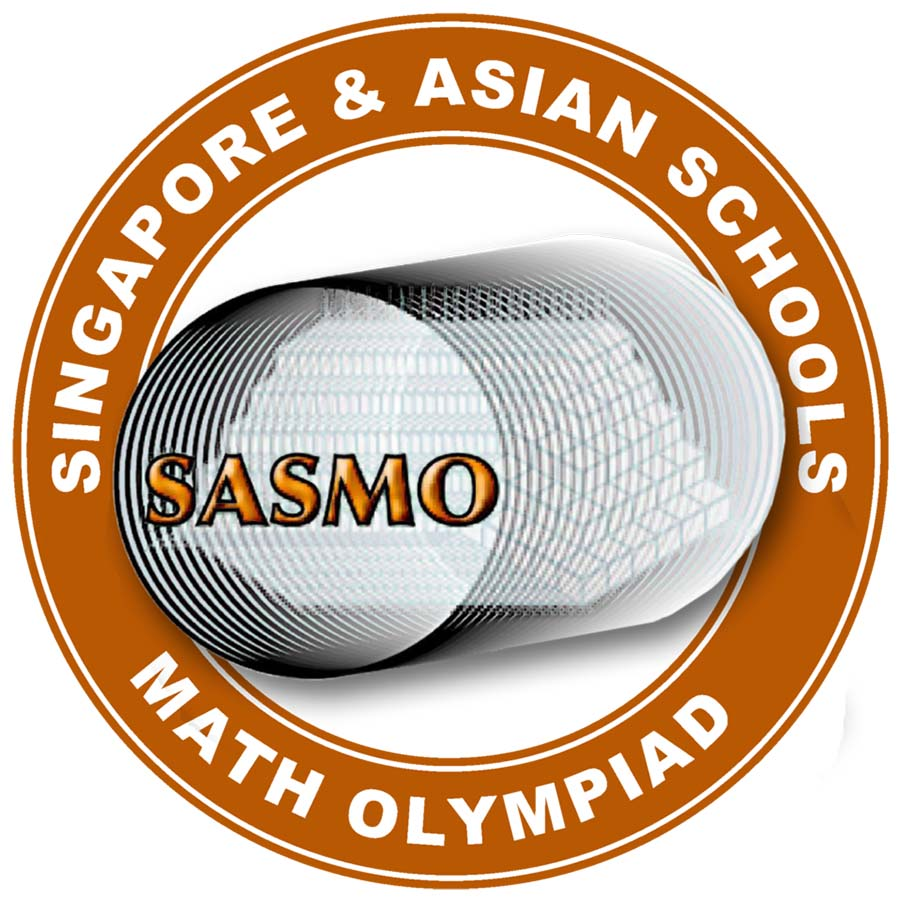Singapore and Asian Schools Math Olympiad (SASMO) is an international mathematics competition that is organised by Singapore International Math Contests Centre (SIMCC) and supported by non-profit foundations Singapore Scholastic Trust and SASMO Advisory Council (SAC).

• April every year

### Class 2 (Primary 2)

Time, Addition and Subtraction, Multiplication, Number Pattern, Counting, Shortest Path, Queuing Problem, Pigeonhole Principle, IQ, Logic, Odd and Even Numbers, Sum of a Number Sequence, Working Backwards, Simple Speed

### Class 3 (Primary 3)

Day and Time, Addition and Subtraction, Multiplication, Division, Remainder Problem, Number Pattern, Counting, Shortest Path, Queuing Problem, Pigeonhole Principle, Logic, Odd and Even Numbers, Sum of a Number Sequence, Working Backwards, Speed, Value of Ones Digit

### Class 4 (Primary 4)

Time, Addition and Subtraction, Multiplication, Division, Remainder Problem, Number Pattern, Number Puzzle, Counting, Queuing Problem, Pigeonhole Principle, IQ, Logic, Odd and Even Numbers, Sum of a Number Sequence, Working Backwards, Speed, Value of Ones Digit

### Class 5 (Primary 5)

The Four Operations, Whole Numbers, Fractions, Permutation and Combination, Pigeonhole Principle, Number Sequence, Logic, Average, Square Number, Prime Numbers, Prime factorization, Defining New Operation, Working Backwards, Speed, Ratio and Percentages, Area of Circle, Angle and Triangles, Probability, Charts

### Class 6 (Primary 6)

The Four Operations, Whole Numbers, Fractions, Permutation and Combination, Pigeonhole Principle, Number Sequence, Logic, Average, Square Number, Prime Numbers, Prime factorization, Defining New Operation, Working Backwards, Speed, Ratio and Percentages, Area of Circle, Angle and Triangles, Probability, Charts

### Class 7 (Secondary 1 )

Simple Equations, Rational Numbers, Indices, System of Equations, Multiplication of Polynomials, Divisiblity, Prime and Composite Numbers, Square Numbers, Even and Odd Numbers, GCD and LCM, Permutation and Combination, Probability, Pythagorean Theorem, Pigeonhole Principle, Problem on Journey, Profit and Loss, Circles, Geometry

### Class 8 (Secondary 2 )

Simple Equations, Rational Numbers, Indices, System of Equations, Multiplication of Polynomials, Divisiblity, Prime and Composite Numbers, Square Numbers, Even and Odd Numbers, GCD and LCM, Permutation and Combination, Probability, Pythagorean Theorem, Pigeonhole Principle, Problem on Journey, Profit and Loss, Circles, Geometry

### Class 9 (Secondary 3 )

Simple Equations, Rational Numbers, Indices, System of Equations, Polynomials, Divisiblity, Prime and Composite Numbers, Square Numbers, Even and Odd Numbers, GCD and LCM, Permutation and Combination, Probability, Pythagorean Theorem, Pigeonhole Principle, Journey Problem, Profit and Loss, Circles, Geometry

### Class 10 (Secondary 4 )

Simple Equations, Rational Numbers, Indices, System of Equations, Polynomials, Divisiblity, Prime and Composite Numbers, Square Numbers, Even and Odd Numbers, GCD and LCM, Permutation and Combination, Probability, Pythagorean Theorem, Pigeonhole Principle, Journey Problem, Profit and Loss, Circles, Geometry